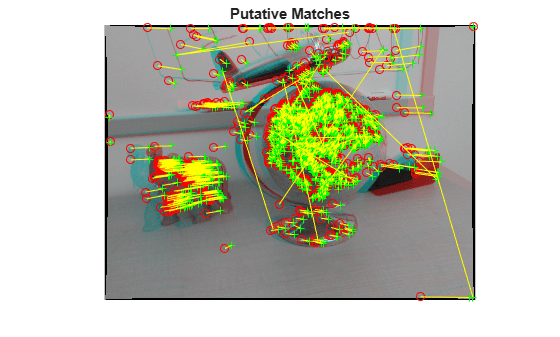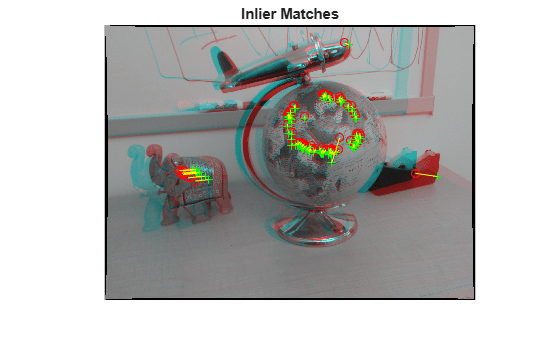# estimateEssentialMatrix

Estimate essential matrix from corresponding points in a pair of images

## Syntax

``E = estimateEssentialMatrix(matchedPoints1,matchedPoints2,cameraParams)``
``E = estimateEssentialMatrix(matchedPoints1,matchedPoints2,cameraParams1,cameraParams2)``
``````[E,inliersIndex] = estimateEssentialMatrix(___)``````
``````[E,inliersIndex,status] = estimateEssentialMatrix(___)``````
``````[___] = estimateEssentialMatrix(___,Name,Value)``````

## Description

example

````E = estimateEssentialMatrix(matchedPoints1,matchedPoints2,cameraParams)` returns the 3-by-3 essential matrix, `E`, using the M-estimator sample consensus (MSAC) algorithm. The input points can be M-by-2 matrices of M number of [x,y] coordinates, or a `KAZEPoints` , `SIFTPoints`,`SURFPoints`, `MSERRegions`, `BRISKPoints`, or `cornerPoints` object. The `cameraParams` object contains the parameters of the camera used to take the images.```
````E = estimateEssentialMatrix(matchedPoints1,matchedPoints2,cameraParams1,cameraParams2)` returns the essential matrix relating two images taken by different cameras. `cameraParams1` and `cameraParams2` are `cameraParameters` objects containing the parameters of camera 1 and camera 2 respectively.```
``````[E,inliersIndex] = estimateEssentialMatrix(___)``` additionally returns an M-by-1 logical vector, `inliersIndex`, used to compute the essential matrix. The function sets the elements of the vector to `true` when the corresponding point was used to compute the fundamental matrix. The elements are set to `false` if they are not used.```
``````[E,inliersIndex,status] = estimateEssentialMatrix(___)``` additionally returns a status code to indicate the validity of points.```
``````[___] = estimateEssentialMatrix(___,Name,Value)``` uses additional options specified by one or more Name,Value pair arguments.```

## Examples

collapse all

`load upToScaleReconstructionCameraParameters.mat`

```imageDir = fullfile(toolboxdir('vision'),'visiondata',... 'upToScaleReconstructionImages'); images = imageDatastore(imageDir); I1 = undistortImage(readimage(images,1),cameraParams); I2 = undistortImage(readimage(images,2),cameraParams); I1gray = im2gray(I1); I2gray = im2gray(I2);```

Detect feature points each image.

```imagePoints1 = detectSURFFeatures(I1gray); imagePoints2 = detectSURFFeatures(I2gray);```

Extract feature descriptors from each image.

```features1 = extractFeatures(I1gray,imagePoints1,'Upright',true); features2 = extractFeatures(I2gray,imagePoints2,'Upright',true);```

Match features across the images.

```indexPairs = matchFeatures(features1,features2); matchedPoints1 = imagePoints1(indexPairs(:,1)); matchedPoints2 = imagePoints2(indexPairs(:,2)); figure showMatchedFeatures(I1,I2,matchedPoints1,matchedPoints2); title('Putative Matches')```Estimate the essential matrix.

```[E,inliers] = estimateEssentialMatrix(matchedPoints1,matchedPoints2,... cameraParams);```

Display the inlier matches.

```inlierPoints1 = matchedPoints1(inliers); inlierPoints2 = matchedPoints2(inliers); figure showMatchedFeatures(I1,I2,inlierPoints1,inlierPoints2); title('Inlier Matches')```## Input Arguments

collapse all

Coordinates of corresponding points in image 1, specified as an M-by-2 matrix of M of [x,y] coordinates, or as a `KAZEPoints`, `SIFTPoints`, `SURFPoints`, `BRISKPoints`, `MSERRegions`, or `cornerPoints` object. The `matchedPoints1` input must contain at least five points, which are putatively matched by using a function such as `matchFeatures`.

Coordinates of corresponding points in image 1, specified as an M-by-2 matrix of M of [x,y] coordinates, or as a `KAZEPoints`, `SIFTPoints`, `SURFPoints`, `MSERRegions`, `BRISKPoints`,or `cornerPoints` object. The `matchedPoints2` input must contain at least five points, which are putatively matched by using a function such as `matchFeatures`.

Camera parameters, specified as a `cameraParameters` or `cameraIntrinsics` object. You can return the `cameraParameters` object using the `estimateCameraParameters` function. The `cameraParameters` object contains the intrinsic, extrinsic, and lens distortion parameters of a camera.

Camera parameters for camera 1, specified as a `cameraParameters` or `cameraIntrinsics` object. You can return the `cameraParameters` object using the `estimateCameraParameters` function. The `cameraParameters` object contains the intrinsic, extrinsic, and lens distortion parameters of a camera.

Camera parameters for camera 2, specified as a `cameraParameters` or `cameraIntrinsics` object. You can return the `cameraParameters` object using the `estimateCameraParameters` function. The `cameraParameters` object contains the intrinsic, extrinsic, and lens distortion parameters of a camera.

### Name-Value Arguments

Specify optional pairs of arguments as `Name1=Value1,...,NameN=ValueN`, where `Name` is the argument name and `Value` is the corresponding value. Name-value arguments must appear after other arguments, but the order of the pairs does not matter.

Before R2021a, use commas to separate each name and value, and enclose `Name` in quotes.

Example: `'MaxNumTrials'`, `500`

Maximum number of random trials for finding outliers, specified as the comma-separated pair consisting of '`MaxNumTrials`' and a positive integer. The actual number of trials depends on `matchedPoints1`, `matchedPoints2`, and the value of the `Confidence` parameter. To optimize speed and accuracy, select the number of random trials.

Desired confidence for finding the maximum number of inliers, specified as the comma-separated pair consisting of '`Confidence`' and a percentage scalar value in the range (0,100). Increasing this value improves the robustness of the output but increases the amount of computations.

Sampson distance threshold, specified as the comma-separated pair consisting of '`MaxDistance`' and a scalar value. The function uses the threshold to find outliers returned in pixels squared. The Sampson distance is a first-order approximation of the squared geometric distance between a point and the epipolar line. Increase this value to make the algorithm converge faster, but this can also adversely affect the accuracy of the result.

## Output Arguments

collapse all

Essential matrix, returned as a 3-by-3 matrix that is computed from the points in the `matchedPoints1` and `matchedPoints2` inputs with known camera intrinsics.

`$\left[\begin{array}{cc}{P}_{2}& 1\end{array}\right]*EssentialMatrix*{\left[\begin{array}{cc}{P}_{1}& 1\end{array}\right]}^{\text{'}}=0$`

The P1 point in image 1, in normalized image coordinates, corresponds to the , P2 point in image 2.

In computer vision, the essential matrix is a 3-by-3 matrix which relates corresponding points in stereo images which are in normalized image coordinates. When two cameras view a 3-D scene from two distinct positions, the geometric relations between the 3-D points and their projections onto the 2-D images lead to constraints between image points. The two images of the same scene are related by epipolar geometry.

Data Types: `double`

Inliers index, returned as an M-by-1 logical index vector. An element set to `true` indicates that the corresponding indexed matched points in `matchedPoints1` and `matchedPoints2` were used to compute the essential matrix. An element set to `false` means the indexed points were not used for the computation.

Data Types: `logical`

Status code, returned as one of the following possible values:

statusValue
`0`:No error.
`1`:`matchedPoints1` and `matchedPoints2` do not contain enough points. At least five points are required.
`2`:Not enough inliers found. A least five inliers are required.

Data Types: `int32`

## Tips

Use `estimateEssentialMatrix` when you know the camera intrinsics. You can obtain the intrinsics using the Camera Calibrator app. Otherwise, you can use the `estimateFundamentalMatrix` function that does not require camera intrinsics. The fundamental matrix cannot be estimated from coplanar world points.

 Kukelova, Z., M. Bujnak, and T. Pajdla Polynomial Eigenvalue Solutions to the 5-pt and 6-pt Relative Pose Problems. Leeds, UK: BMVC, 2008.

 Nister, D.. “An Efficient Solution to the Five-Point Relative Pose Problem.” IEEE Transactions on Pattern Analysis and Machine Intelligence.Volume 26, Issue 6, June 2004.

 Torr, P. H. S., and A. Zisserman. “MLESAC: A New Robust Estimator with Application to Estimating Image Geometry.” Computer Vision and Image Understanding. Volume 78, Issue 1, April 2000, pp. 138-156.